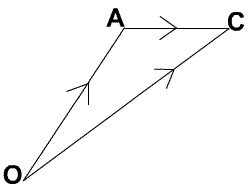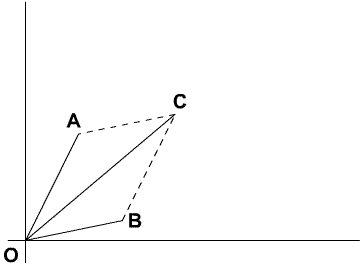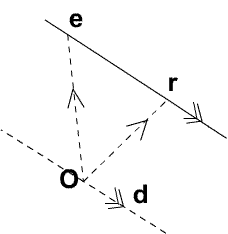# An Introduction to Vectors

##### Age 14 to 18

Published June 2004,February 2011.

This article describes what vectors are and how to add, subtract and multiply them by scalars, and it gives some indications of why they are useful. The article provides a summary of the elementary ideas about vectors usually met in school mathematics. The follow-up article 'Multiplication of Vectors' discusses scalar products and vector products. Vectors are an absolutely essential 'tool' in physics and a very important part of mathematics.

There are two ways to define vectors. We can think of vectors as points in a coordinate system corresponding to points in space, or we can think of vectors as objects with magnitude and direction. In this article we attempt to clarify why there are two definitions of vectors and relate the two. The most perceptive and mathematically able school students often feel they don't understand the use of vectors and they are absolutely right to question this because school textbooks often switch between the different sorts of vectors without justifying what they are doing.

Vectors are usually first introduced as objects having magnitude and direction, for example translations, displacements, velocities, forces etc. Vectors defined this way are called free vectors . If we simply specify magnitude and direction then any two vectors of the same length and parallel to each other are considered to be identical. So by this definition a vector is an infinite set of parallel directed line segments.

For example consider the translation three units across and one unit up. If we apply this translation then the point $(0, 0)$ goes to $(3, 1)$, and $(5, 7)$ goes to $(8, 8)$, and every other point is translated similarly. You can draw your own diagrams to illustrate this. A translation of the plane moves all the points of the plane simultaneously by the same translation vector, so we can think of a free vector as the same thing as a translation.

People often choose one line segment from this infinite set to suit a particular application and it is sensible to ask why in practice we can take a single representative without reference to the whole set. For example, if we walk from $O$ to $A$ we denote the displacement by $\vec{OA}$. This is different from walking the same distance in a different direction, and different from walking in the same direction but going a different distance. Some people call this displacement the vector $\vec{OA}$ but we are thinking of $O$ as a particular point so this is one directed line segment chosen from the whole set which constitute the free vector. If we continue our walk from $A$ to $C$ the total displacement is the sum of these two displacements $\vec{OA}+\vec{AC}$ and this is equal to the directed line segment $\vec{OC}$. The triangle law is used to add free vectors.Vectors are important in navigation where the actual velocity of an aeroplane relative to the earth is given by the combined velocities of the wind (which carries the plane along as if it were a glider) together with the velocity which the plane would have in still air. In the triangle above, if $\vec{OC}$ is along the direction required to reach the destination, and $\vec{AC}$ represents the velocity of the wind, then the pilot has to set a course in the direction $\vec{OA}$ with a speed calculated so that the sides of the triangle represent the velocities.

In mathematics we think of points and space as fundamental abstract concepts and we build a model of space by using a coordinate system. A three dimensional coordinate system is simply an infinite set of ordered triples of real numbers $(x, y, z)$ and each point is given by one of these ordered triples, called the coordinates of the point. To each free vector (or translation) there corresponds a position vector which is the image of the origin under that translation. So we define position vectors as points in space and to each position vector $P$ there corresponds a directed line segment $\vec {OP}$ which determines an infinite set of parallel directed line segments giving a unique free vector.

When we choose a coordinate system we effectively single out one representative from each free vector in space, namely the one which 'starts' from the chosen origin. If the point $A$ has coordinates $(x_a, y_a, z_a)$ then it has position vector ${\bf a} = (x_a, y_a, z_a)$ which may also be written as a column vector. There is a correspondence between the point $A$, the position vector ${\bf a}$ and the directed line segment $\vec {OA}$ which is a representative of the infinitely many segments making up a free vector.

It is frequently useful to work only with position vectors and not with free vectors. While there is a conceptual distinction between free vectors and position vectors it is possible to use both types interchangeably but this may cause confusion if we are not clear about the definitions.

All the vector algebra (adding, subtracting, multiplying) which works in one system corresponds to the vector algebra in the other system. When it suits us to do so we can switch from free vectors to position vectors or vice versa, do the vector algebra, then switch back with 'the answer'.

The magnitude of the position vector ${\bf a}=(x_a, y_a, z_a)$ is defined to be $$|{\bf a}|= (x_a^2+y_a^2+z_a^2)^{1/2}$$ and this is the length of the line segment $\vec{OA}$ and hence it is also the magnitude of the corresponding free vector.

The vectors ${\bf i}= (1, 0, 0)$, ${\bf j}=(0, 1, 0)$ and ${\bf k}=(0, 0, 1)$ are vectors of unit length parallel to the $x, y$ and $z$ axes. The position vector or point $A$ and the corresponding free vector consisting of all directed line segments parallel to $\vec {OA}$ can also be written as $x_a{\bf i}+y_a{\bf j}+z_a{\bf k}$.

Some elementary textbooks say that forces are vectors but are they? Strictly speaking they are a special type of vector with more structure than other vectors; as well as magnitude and direction forces are specified by their point or line of action. If I push your right shoulder hard enough you will turn one way and if I push your left shoulder with a force of the same magnitude in the same direction (an equal vector) you will turn the other way. The two forces have different turning effects so they are different forces even though they have the same 'vector properties'. When we add forces we simply use their vector properties but to specify a force we need to give its magnitude, direction and line of action.

#### Addition and subtraction of vectors

To add position vectors we simply add the components. For example if $\bf a$ is the position vector $(x_a, y_a, z_a)$ and $\bf b$ is the position vector $(x_b, y_b, z_b)$ then ${\bf a} + {\bf b} = (x_a+x_b,\ y_a+y_b,\ z_a+z_b).$ The parallelogram law is used to add position vectors giving $\vec{OA}+\vec{OB}=\vec{OC}$.Note that, as a free vector ${\vec {OB}} = {\vec {AC}}$ so the parallelogram law of addition of position vectors exactly corresponds to the triangle law, ${\vec {OA}}+ {\vec {AC}} = {\vec {OC}}$, of addition of free vectors and hence they can be used interchangeably for either type of vector.

What about subtraction? Each point $A$ in space is a vector with components the same as the coordinates of the point, say ${\bf a}=(x_a,y_a,z_a)$. The reflection of the point $A$ in the origin is the point $A'$ with position vector $-{\bf a}=(-x_a,-y_a,-z_a)$. The effect of adding these two vectors is to give the zero vector. To subtract the vector ${\bf a}$ from the vector ${\bf c}$ simply add the vectors ${\bf c}$ and ${\bf -a}$.

The directed line segments $\vec{OA}$ and $\vec{OA'}$ are equal in length and opposite in direction so we say $\vec{OA'}=-\vec{OA}$. The equivalent method of subtraction for free vectors can be thought of as reversing the vector to be subtracted and adding it to the first vector. If $A$ and $C$ are two points $(x_a, y_a, z_a)$ and $(x_c, y_c, z_c)$ then the directed line segment ${\vec{OC}}= {\vec{OA}} + {\vec{AC}}$ so again we see that to subtract vectors we subtract the components. $${\vec {AC}}= {\vec {OC}} - {\vec {OA}} = \left( \begin{array}{cc} x_c-x_a\\ y_c-y_a\\ z_c-z_a \end{array} \right)$$

#### Multiplication of a vector by a scalar

We have already seen scalar multiples when we wrote $(x_a, y_a, z_a) = x_a{\bf i}+y_a{\bf j}+z_a{\bf k}$. Here the vectors ${\bf i}, {\bf j}$ and ${\bf k}$ are multiplied by the scalars : $x_a, y_a$ and $z_a$.One of the uses of multiplication of vectors by scalars is to write down an equation of a line using vectors. If a vector ${\bf d}$ is along a line then any other vector along the line is a multiple of ${\bf d}$ and we can call ${\bf d}$ a direction vector for the line. In writing down the equation of a line we use the notation ${\bf r} = (x,y,z)$ for the vector of a general point on the line, ${\bf e}=(x_1, y_1, z_1)$ for the vector of one particular point known to be on the line, $s$ as a scalar variable and ${\bf d} = (l,m,n)$ for a direction vector along the line. The vector equation of the line is then ${\bf r} = {\bf e} + s{\bf d}.$

As we have seen there are two distinct types of vectors but it is permissable to switch from one to the other when convenient to do so. The follow up article 'Multiplication of Vectors' completes the summary of the basic ideas in the subject.